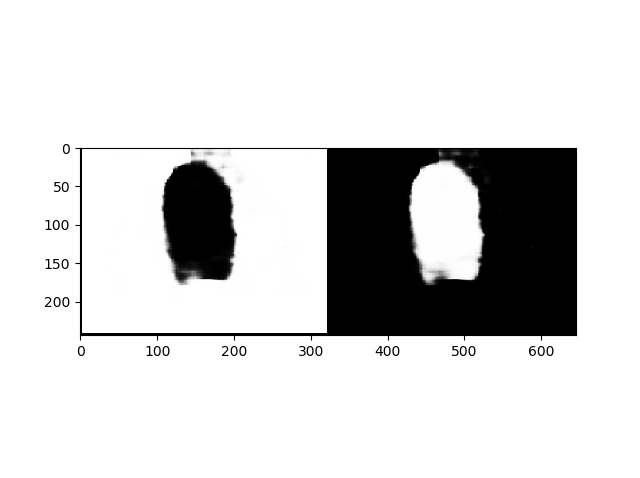# How can I display only the edges in semantic segmentation?

The model could predict the classes as shown in the attached image; I was wondering how is it possible to display only the edges of the predicted objcet?
the image is the probability map of output.

``````    outputs = model(t_image)
plt.figure()
prob = torch.exp(outputs)
prob_imgs = make_grid(prob.permute(1, 0, 2, 3))
prob_imgs = prob_imgs.detach()
plt.imshow(prob_imgs.permute(1, 2, 0))
plt.show()
``````Hi @Neda, maybe try binarizing the output?

An edge detector can also be applied to the image.
http://scikit-image.org/docs/dev/auto_examples/edges/plot_canny.html

1 Like

@Antonio_Rueda_Toicen Thank you for the link. I will have a look.